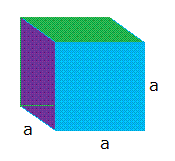# Surface Area of a Cube

To derive the formula of the surface area of a cube, you will need to start with a cube as shown below and call the length of one side a.In order to make a cube like the one shown above, you basically use the following cube template:Looking at the cube template, it is easy to see that the cube has six sides and each side is a square

The area of one square is a × a = a2

Since there are six sides, the total surface area, call it SA is:

SA = a2 + a2 + a2 + a2 + a2 + a2

SA = 6 × a2

Example #1:

Find the surface area if the length of one side is 3 cm

Surface area = 6 × a2

Surface area = 6 × 32

Surface area = 6 × 3 × 3

Surface area = 54 cm2

Example #2:

Find the surface area if the length of one side is 5 cm

Surface area = 6 × a2

Surface area = 6 × 52

Surface area = 6 × 5 × 5

Surface area = 150 cm2

Example #3:

Find the surface area if the length of one side is 1/2 cm

Surface area = 6 × a2

Surface area = 6 × (1/2)2

Surface area = 6 × 1/2 × 1/2

Surface area = 6 × 1/4

Surface area = 6/4 cm2

Surface area = 3/2 cm2

Surface area = 1.5 cm2